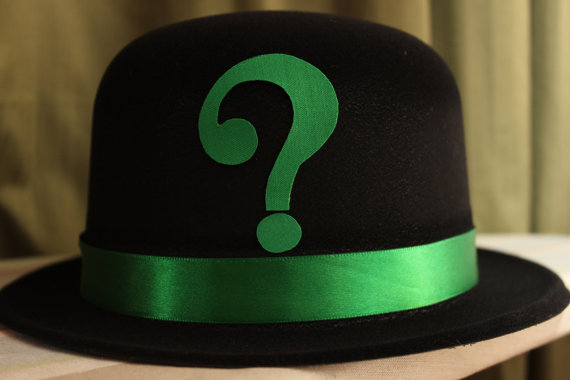# The Ultimate Hat Challenge

Logic Level 3Calvin, Brian, and Jon each wear a hat with a positive integer, and they are told that the number on one of the hats is the sum of the numbers on the other two. Each person can see the numbers on the hats other than their own. The following conversation ensues:

• Calvin: I don't know my number.
• Brian: I don't know my number.
• Jon: I don't know my number.
• Calvin: My number is 50.

What are the numbers on Brian's hat and Jon's hat?

Concatenate the two answers, with Brian's number first. For example, if you think Brian's and Jon's numbers are 90 and 40 respectively, write the answer as 9040.

×